# Questions on Inverse Functions with Solutions

Analytical and graphing methods are used to solve maths problems and questions related to inverse functions. Detailed solutions are also presented. Several questions involve the use of the property that the graphs of a function and the graph of its inverse are reflection of each other on the line y = x.

1. Question
Below is shown the graph of f(x) = 2 x 3 - 1.

1) Sketch the graph of the inverse of f in the same system of axes.
2) Find the inverse of and check your answer using some points.
Solution
1) Locate few points on the graph of f. Here is a list of points whose coordinates (a , b) can easily be determined from the graph:
(1 , 1) , (0 , -1) , (-1 , -3)
On the graph of the inverse function, the above points will have coordinates (b , a) as follows:
(1 , 1) , (-1 , 0) , (-3 , -1)
Plot the above points and sketch the graph of the inverse of f so that the two graphs are reflection of each other on the line y = x as shown below.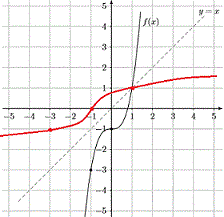.

2) Write the given function f(x) = 2 x 3 - 1 as an equation in two unknowns.
y = 2 x 3 - 1
Solve the above for x.
2 x 3 = y + 1
x 3 = (y + 1) / 2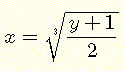Interchange x and y and write the equation of inverse function f -1:.
We now verify that the points (1 , 1) , (-1 , 0) and (-3 , -1) used above to sketch the graph of the inverse function are on the graph of f -1.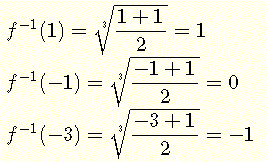.

2. Question
Let f(x) = x 2 - 4 x + 5, x ≤ 2.
1) Find the inverse function of f.
2) Find the domain and the range of f -1.
Solution
1) We are given a quadratic function with a restricted domain. We first write the given function in vertex form (may be done by completing the square):
f(x) = x 2 - 4 x + 5 = (x - 2) 2 + 1 , x ≤ 2
The graph of function f is that of the left half of a parabola with vertex at (2 , 1) as shown below.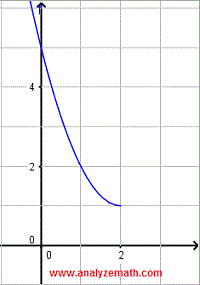.

We now write the given function as an equation.
y =(x - 2) 2 + 1
Solve the above for x.
y =(x - 2) 2 + 1
(x - 2) 2 = y - 1
Two solutions for x - 2:     x - 2 = +√(y - 1)     or     x - 2 = - √(y - 1)
x = √(y - 1) + 2     or     x = - √(y - 1) + 2
Since x ≤ 2 (domain of f), we select the solution
x = - √(y - 1) + 2
Interchange x and y to write the inverse of function f as follows.
y = f -1(x) = - √(x - 1) + 2
The domain and range of f -1 are the range and domain of f.
Domain of f -1 is the range of f: [1 , +∞)     (from graph)
Range of f -1 is the domain of f: (-∞ , 2]     (given)

3. Question
Below is shown the graph of f(x) = √(2 x - 3).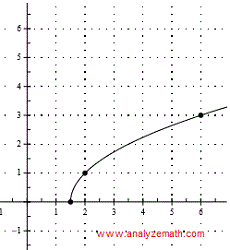.

1) Sketch the inverse of f in the same graph.
2) Find the inverse of and check your answer using some points.
Solution
1) Locate few points on the graph of f. A possible list of points whose coordinates (a , b) is as follows:
(1.5 , 0) , (2 , 1) , (6 , 3)
On the graph of the inverse function, the above points will have coordinates (b , a) as follows:
(0 , 1.5) , (1 , 2) , (3 , 6)
Plot the above points and sketch the graph of the inverse of f so that the two graphs are reflection of each other on the line y = x as shown below.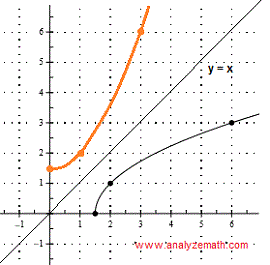.

2) Write the given function f(x) = √(2 x - 3) as an equation in two unknowns.
y = √(2 x - 3)
Solve the above for x. First square both sides
2 x - 3 = y 2
2 x = y 2 + 3
x = (y 2 + 3) / 2
Interchange x and y and write the equation of the inverse function f -1; and write the domain of the inverse.
y = (x 2 + 3) / 2
f -1 (x) = (x 2 + 3) / 2 , x ≥ 0 (domain which is the range of f from its graph above)
We now verify that the points (0 , 1.5) , (1 , 2) and (3 , 6) used to sketch the graph of the inverse function are on the graph of f -1.
f -1(0) = (0 2 + 3) / 2 = 1.5
f -1(1) = (1 2 + 3) / 2 = 2
f -1(3) = (3 2 + 3) / 2 = 6

4. Question
Sketch the graph of f -1 using the graph of y = f(x) shown below and find f -1(x).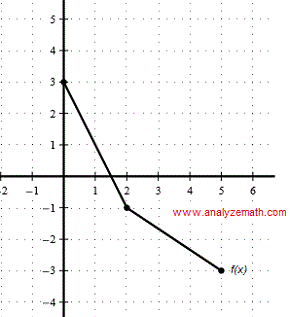.

Solution
1) Use the graph to find points on the graph of f. A possible list of points whose coordinates (a , b) is as follows:
(0 , 3) , (2 , -1) , (5 , - 3)
On the graph of the inverse function, the above points will have coordinates (b , a) as follows:
(3 , 0) , (- 1 , 2) , (- 3 , 5)
Plot the above points and sketch the graph of the inverse of f so that the two graphs are reflection of each other on the line y = x as shown below.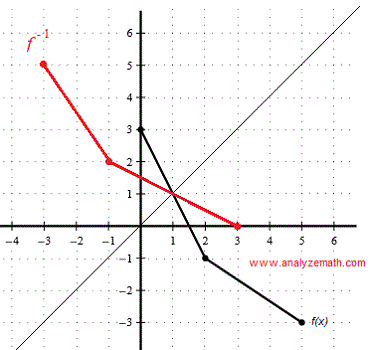.

2) We now determine f -1(x). For -3 ≤ x ≤ - 1 , f -1(x) has a linear expression with slope m1 through the points (- 1 , 2) , (- 3 , 5) given by
m1 = (5 - 2) / (-3 - (-1)) = - 3 / 2
For -3 ≤ x ≤ - 1, f -1(x) is given by:
f -1(x) = - (3 / 2)(x - (-1)) + 2 = - (3 / 2)(x + 1) + 2
For - 1 < x ≤ 3 , f -1(x) has a linear expression with slope through the points (- 1 , 2) , (3 , 0) given by
m2 = (0 - 2) / (3 - (-1)) = - 1 / 2
For - 1 < x ≤ 3, f -1(x) is given by:
f -1(x) = - (1 / 2)(x - (-1)) + 2 = - (1 / 2)(x + 1) + 2

5. Question
The one to one function. is graphed below..
1) What is the domain and range of f?
2) Sketch the graph of f -1.
3) Find f -1(x) (include domain).
Solution
1) f(x) is defined as a real number if the radicand 2 / x - 1 is greater than or equal to 0. Hence we need to solve the inequality:
2 / x - 1 ≥ 0
(2 - x) / x ≥ 0
The expression on the left of the inequality changes sign at the zeros of the numerator and denominator which are x = 2 and x = 0. See table below..

Domain: (0 , 2]
Range: (-∞ , 0]
2) Points on the graph of f
(2 , 0) , (1 , -1)
The above points on the graph of the inverse function, will have coordinates (b , a) as follows:
(0 , 2) , (- 1 , 1)
Plot the above points and sketch the graph of the inverse of f so that the two graphs are reflection of each other on the line y = x as shown below..

3) Write f(x) as an equation in y and x.
$$y = -\sqrt{\dfrac{2}{x}-1}$$
Solve the above equation for x. Square both sides of the above equation
$$y^2 = \dfrac{2}{x}-1$$
$$\dfrac{2}{x} = y^2 + 1$$
$$x = \dfrac{2}{y^2 + 1}$$
Interchange x and y and write the inverse function
$$y = \dfrac{2}{x^2 + 1}$$
$$f^{-1}(x) = \dfrac{2}{x^2 + 1}$$
Domain and range of f-1 are the range and domain of f . Hence
Domain of f -1: (-∞ , 0]
Range of f -1: (0 , 2]

6. Question
Below are shown the graph of 6 functions. Sketch the graph of the inverse of each function.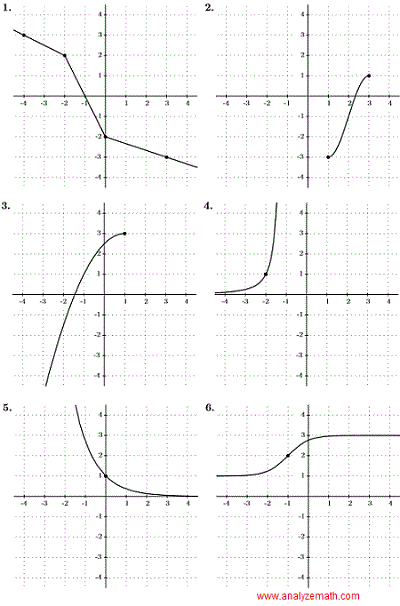.

Solution
For each graph, select points whose coordinates are easy to determine. Use these points and also the reflection of the graph of function f and its inverse on the line y = x to skectch to sketch the inverse functions as shown below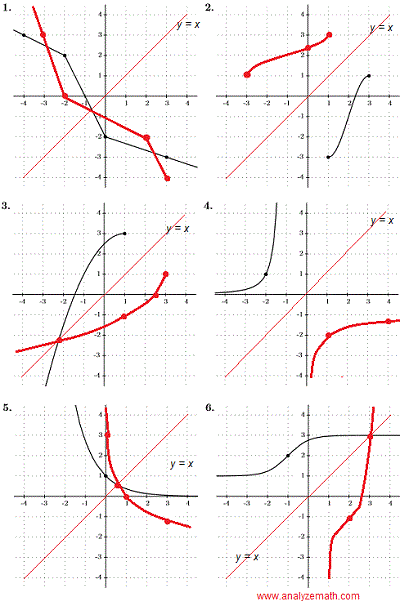.

7. Question
Find the inverse of f(x) = Log4(x + 2) - 5, its domain and range.
Solution
Write the given function as an equation in x and y as follows:
y = Log4(x + 2) - 5
Solve the above equation for x.
Log4(x + 2) = y + 5
x + 2 = 4 (y + 5)
x = 4 (y + 5) - 2
Interchange x and y.
y = 4 (x + 5) - 2
Write the inverse function with its domain and range.
f-1(x) = 4 (x + 5) - 2 , Domain: (-∞ , +∞) , Range: (-2 , +∞)

8. Question
If f(x) = ln(x) + 4 x - 8, what is the value of f -1(- 4)?
Solution
Let a = f -1(- 4). Then
f(a) = f(f -1(- 4)) = - 4 (Using the property f(f -1(x)) = x of the inverse function).
We now need to find a such that f(a) = - 4 hence the equation to solve.
ln(a) + 4 a - 8 = - 4
ln(a) = 4 - 4 a
The above equation cannot be solved analytically but its solution may be approximated graphically as the x coordinate of the point of intersection of the graphs of y = ln(x) and y = 4 - 4x as shown below..

The intersection of the two graphs is close to x = 1 which can easily be checked that it is the exact solution to the equation ln(x) = 4 - 4 x. Hence
f-1( - 4) = 1